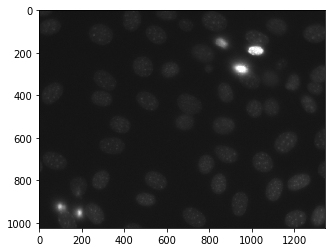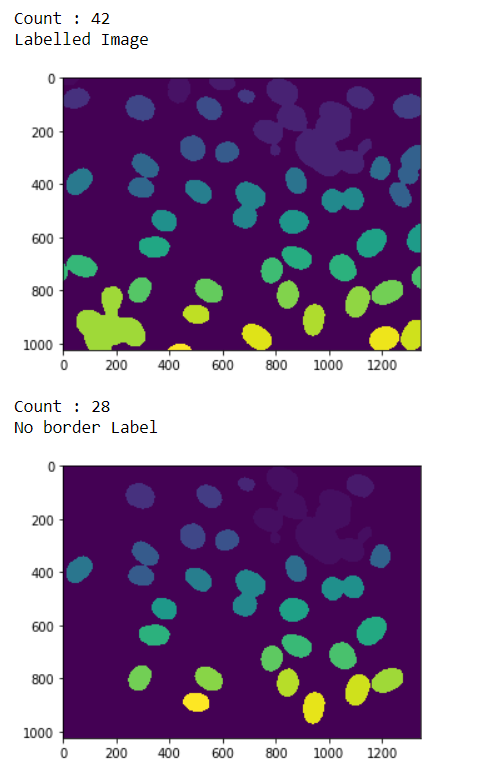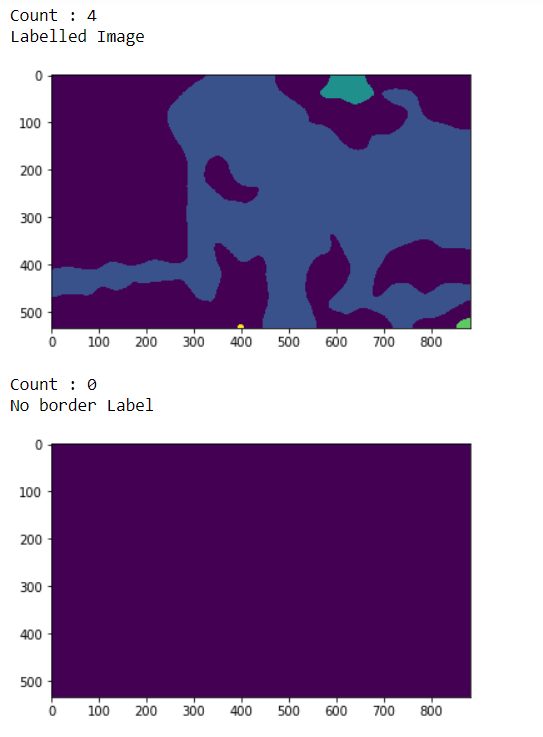Related Articles

# Mahotas – Re-Labeling

• Last Updated : 22 Apr, 2021

In this article we will see how we can relabel the labelled image in mahotas. Relabeling is used to label the already labelled image, this is required because some times there are mane labels which user deletes so when that image get relabel, we get the new label number as well. We use mahotas.label method to label the image
For this we are going to use the fluorescent microscopy image from a nuclear segmentation benchmark. We can get the image with the help of command given below

`mhotas.demos.nuclear_image()`

Below is the nuclear_imageLabelled images are integer images where the values correspond to different regions. I.e., region 1 is all of the pixels which have value 1, region two is the pixels with value 2, and so on
In order to do this we will use mahotas.relabel method

Syntax : mahotas.relabel(labelled)
Argument : It takes labelled image object as argument
Return : It returns the labelled image and integer i.e number of labels

Example 1:

## Python3

 `# importing required libraries``import` `mahotas``import` `numpy as np``from` `pylab ``import` `imshow, show``import` `os` `# loading nuclear image``f ``=` `mahotas.demos.load(``'nuclear'``)` `# setting filter to the image``f ``=` `f[:, :, ``0``]` `# setting gaussian filter``f ``=` `mahotas.gaussian_filter(f, ``4``)` `# setting threshold value``f ``=` `(f> f.mean())` `# creating a labelled image``labelled, n_nucleus ``=` `mahotas.label(f)` `# printing number of labels``print``(``"Count : "` `+` `str``(n_nucleus))` `# showing the labelled image``print``(``"Labelled Image"``)``imshow(labelled)``show()` `# removing border labels``labelled ``=` `mh.labelled.remove_bordering(labelled)` `# relabling the labelled image``relabelled, n_left ``=` `mahotas.labelled.relabel(labelled)` `# showing number of labels``print``(``"Count : "` `+` `str``(n_left))` `# showing the image``print``(``"No border Label"``)``imshow(relabelled)``show()`

Output :Example 2:

## Python3

 `# importing required libraries``import` `numpy as np``import` `mahotas``from` `pylab ``import` `imshow, show` `# loading image``img ``=` `mahotas.imread(``'dog_image.png'``)``  ` `# filtering the image``img ``=` `img[:, :, ``0``]``   ` `# setting gaussian filter``gaussian ``=` `mahotas.gaussian_filter(img, ``15``)` `# setting threshold value``gaussian ``=` `(gaussian > gaussian.mean())` `# creating a labelled image``labelled, n_nucleus ``=` `mahotas.label(gaussian)` `# printing number of labels``print``(``"Count : "` `+` `str``(n_nucleus))`` ` `print``(``"Labelled Image"``)``# showing the gaussian filter``imshow(labelled)``show()` `# removing border labels``labelled ``=` `mh.labelled.remove_bordering(labelled)` `# relabling the labelled image``relabelled, n_left ``=` `mahotas.labelled.relabel(labelled)` `# showing number of labels``print``(``"Count : "` `+` `str``(n_left))` `# showing the image``print``(``"No border Label"``)``imshow(relabelled)``show()`

Output :Attention geek! Strengthen your foundations with the Python Programming Foundation Course and learn the basics.

To begin with, your interview preparations Enhance your Data Structures concepts with the Python DS Course. And to begin with your Machine Learning Journey, join the Machine Learning – Basic Level Course

My Personal Notes arrow_drop_up